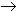#Interactive Real Analysis

Next | Previous | Glossary | Map

## 3.1. Sequences

### Proposition 3.1.6: Algebra on Convergent Sequences

Supposeandare converging to a and b, respectively. Then
1. Their sum is convergent to a + b, and the sequences can be added term by term.
2. Their product is convergent to a * b, and the sequences can be multiplied term by term.
3. Their quotient is convergent to a / b, provide that b # 0, and the sequences can be divided term by term (if the denominators are not zero).
4. If anbn for all n, then ab

### Proof:

The proofs of these statements involve the triangle inequality, as well as an occasional trick of adding and subtracting zero, in a suitable form. A proof of the first statement, for example, goes as follows.

Take any> 0. We know that ana, which implies that there exists an integer N1 such that

| an - a | </ 2
if n > N1. Similarly, since bnb there exists another integer N2 such that
| bn - b | </ 2
if n > N2. But then we know that
| (an + bn) - (a + b) | = | (an - a) + (bn - b) || an - a | + | bn - b |
</2 +/2 =if n > max(N1, N2), which proves the first statement.

Proving the second statement is similar, with some added tricks. We know that { bn } converges, therefore there exists an integer N1 such that

| bn | < |b| + 1
if n > N1. We also know that we can find integers N2 and N3 so that
| an - a | </ (|b| + 1)
if n > N2, and
| bn - b | </ (|a| + 1)
if n > N3, because |a| and |b| are some fixed numbers. But then we have:
| an bn - a b | = | an bn - a bn + a bn - a b |
= | bn(an - a) + a (bn - b) || bn| |an - a | + | a | | bn - b |
< (| b | + 1)/ (|b| + 1) + | a |/ (|a| +1) < 2if n > max(N1, N2, N3), which proves the second statement.

The proof of the third statement is similar, so we will leave it as an exercise.

The last statement does require a new trick: we will use a proof by contradiction to get that result:

Assume that anbn for all n, but a > b.
We now need to work out the contradiction: the idea is that since a > b there is some number c such that b < c < a.
```
<----------[b]-------[a]-------->

<----------[b]--[c]--[a]-------->
```
Since an converges to a, we can make the terms of the sequence fall between c and a, and the terms of bn between b and c. But then we no longer have that anbn, which is our contradiction. Now let's formalize this idea:

Let c = (a + b)/2. Then clearly b < c < a (verify!). Choose N1 such that bn < c if n > N1. That works because b < c. Also choose N2 such that an > c if n > N2. But now we have that

bn < c < an
for n > max(N1, N2). That is a contradiction to the original assumption that anbn for all n. Hence it can not be true that a > b, so that the statement is indeed proved.Next | Previous | Glossary | Map